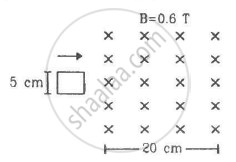Department of Pre-University Education, KarnatakaPUC Karnataka Science Class 12
Advertisement Remove all ads

# Figure Shows a Square Loop of Side 5 Cm Being Moved Towards Right at a Constant Speed of 1 Cm/S. the Front Edge Enters the 20 Cm Wide Magnetic Field at T = 0. - Physics

Sum

Figure shows a square loop of side 5 cm being moved towards right at a constant speed of 1 cm/s. The front edge enters the 20 cm wide magnetic field at t = 0. Find the emf induced in the loop at (a) t = 2 s, (b) t = 10 s, (c) t = 22 s and (d) t = 30 s.Advertisement Remove all ads

#### Solution

Given:-
Initial velocity, u = 1 cm/s
Magnetic field, B = 0.6 T

(a) At t = 2 s:-
Distance moved by the coil = 2 × 1 cm/s = 2 cm = 2 × 10-2m

Area under the magnetic field at t = 2s, A = 2 × 5 × 10-4m2

Initial magnetic flux = 0

Final magnetic flux = BA = 0.6 × (10 × 10-4)T-m2

Change in the magnetic flux, Δϕ = 0.6 × (10 × 10-4) - 0

Now, induced emf in the coil is

$e = \frac{∆ \phi}{∆ t}$

$= \frac{0 . 6 \times (10 - 0) \times {10}^{- 4}}{2}$

$= 3 \times {10}^{- 4} V$

(b) At t = 10 s:-
Distance moved by the coil = 10 × 1 = 10 cm
At this time square loop is completely inside the magnetic field, so there is no change in the flux linked with the coil with time.
Therefore, induced emf in the coil at this time is zero.

(c) At t = 22 s:-
Distance moved = 22 × 1 = 22 cm

At this time loop is moving out of the field.

Initial magnetic flux = 0.6 × (5 × 5 × 10-4)T-m

At this time 2 cm part of the loop is ou t of the field.

Therefore, final magnetic flux = 0.6 × (3 × 5 × 10-4)T-m

Change in the magnetic flux, Δϕ = 0.6 × (3 × 5 × 10-4) - 0.6 × (5 × 5 × 10-4) = - 6 × 10-4)T-m2

Now, induced emf is

$e = \frac{∆ \phi}{∆ t}$

$= \frac{- 6 \times {10}^{- 4}}{2}$

$= - 3 \times {10}^{- 4} V$

(d) At t = 30 s:-
At this time loop is completely out of the field, so there is no change in the flux linked with the coil with time.
Therefore, induced emf in the coil at this time is zero.

Concept: Induced Emf and Current
Is there an error in this question or solution?
Advertisement Remove all ads

#### APPEARS IN

HC Verma Class 11, Class 12 Concepts of Physics Vol. 2
Chapter 16 Electromagnetic Induction
Q 16 | Page 307
Advertisement Remove all ads

#### Video TutorialsVIEW ALL 

Advertisement Remove all ads
Share
Notifications

View all notifications

Forgot password?